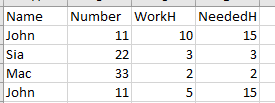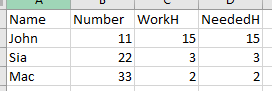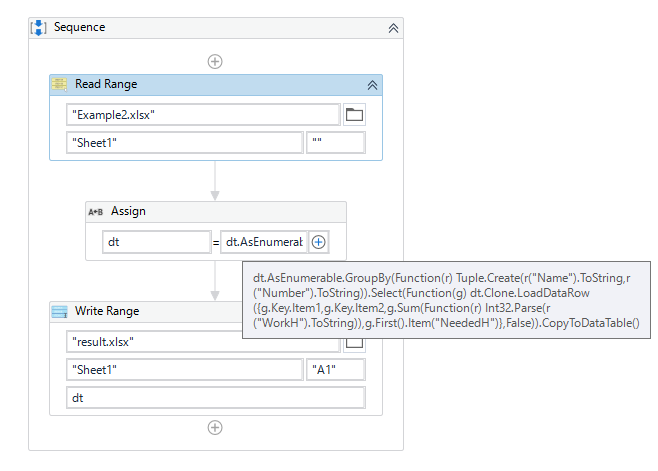# A simple yet frustrating Datatable problem

Hello,
I am having a problem that I just can’t figure out… seems so simple but I can’t get it to work properly so any help would be much appreciated.
Let me explain the problem (a simplified version of course):
I have a data table with 4 columns. Two columns have some sort of ID and the other two have “Work hours” and “Needed Work hours”.
What I need to do is join together the rows that have the same values in the ID columns, copy the “Needed work hours” and Recalculate the “Work hours” by adding together all the rows that apply.

Here is an example DT:So if the “name” and “number” are the same, copy the “Needed Work hours”, and add together the “Work hours”.

Which in this case the result would be something like this:I have tried doing it by making a temporary DT and adding all the same rows and then copying back to the original and adding it together but I can’t seem to get it right.
Here is my workflow… I think I might be on the right path, just can’t quite get it to work…

Also here are some example Datatables for easier testing and development.

Examples.zip (24.7 KB)

Any help would be greatly appreciated!!
Ziga

Hi

Hope the following helps you.`````` dt = dt.AsEnumerable.GroupBy(Function(r) Tuple.Create(r("Name").ToString,r("Number").ToString)).Select(Function(g) dt.Clone.LoadDataRow({g.Key.Item1,g.Key.Item2,g.Sum(Function(r) Int32.Parse(r("WorkH").ToString)),g.First().Item("NeededH")},False)).CopyToDataTable()
``````

Sample20210802-8.zip (27.0 KB)

Regards,

1 Like

Thank you @Yoichi your solution works.
Oddly enough it is slightly slower, with bigger datasets, then my 4 data table solution with two for each loops.
But this is really neat and concise, so I marked it as a solution in case anyone else needs this.1 Like

This topic was automatically closed 3 days after the last reply. New replies are no longer allowed.